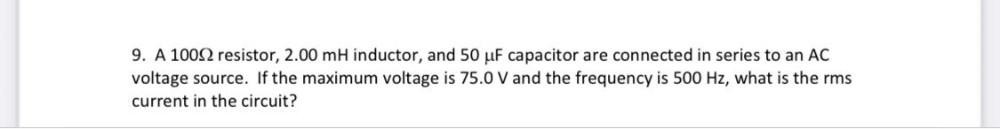Question:

# A 100Ω resistor, 2.00 mH inductor, and 50 µF capacitor are connected in series to an AC voltage source. If the maximum voltage is 75.0 V and the frequency is 500 Hz, what is the rms current in the cirA 100Ω resistor, 2.00 mH inductor, and 50 µF capacitor are connected in series to an AC voltage source. If the maximum voltage is 75.0 V and the frequency is 500 Hz, what is the rms current in the circuit?